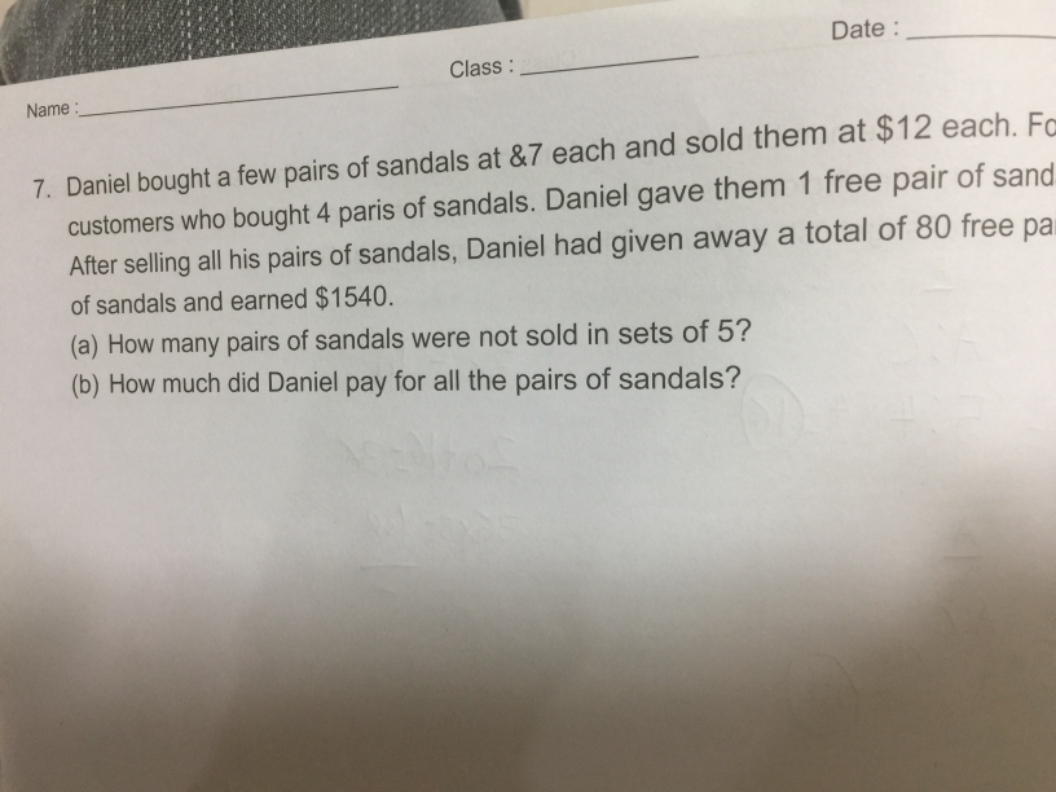# QuestionHi appreciate your help to solve this problem. Thks

He sold 80sets of “buy 4 free 1”

per set cost \$7×5=\$35

per set takings= \$12×4=\$48

profit per set =48-35=\$13

profut in 80 sets = \$13×80=\$1040

balance profit earns from loose pairs = 1540-1040 =\$500

500/(12-7) = 100 pairs of sandals not sold in sets#

total no. of sandals=80×5+100=500

total cost=\$7 x 500=\$3500#

Thks!

0 Replies 0 Likes

(a)
80 x 4 = 320 (paid sandals sold in sets of 4 paid + 1 free pair of sandals)
320 x 12 = 3840 (amount received from sales of 320 paid + 80 free pairs of sandals)
400 x 7 = 2800 (cost of 400 sandals sold in sets of 4 paid + 1 free pair of sandals )
3840 – 2800 = 1040 (amount earned from sales of 320 paid + 80 free pairs of sandals)
1540 – 1140 = 500 (amount earned from sales of sandals not sold in sets of 5)
12 – 7 = 5 (amount earned from sales of each sandal not sold in sets of 5)
500/5 = 100

(b)
400 + 100 = 500
500 x 7 = 3500

Ans : (a) 100 pairs of sandals; (b) \$3500.

0 Replies 1 Like